Technology

# The Kelly Criterion

The Kelly Criterion

The Kelly Criterion

Introduction

So, you’ve probably heard of the Kelly Criterion in the context of gambling or maybe also in the context of investing. When gamblers talk about the Kelly Criterion they are often referring to the idea of sizing your bets, which is the very intuitive idea that if you bet too much of a percentage of your total wealth, you will eventually go bust. The typical example here is that of the confident entrepreneur who wants to sell his house to fund his business, because he knows he has a sure thing.  His wife, who doesn’t know a single thing about Kelly, knows that selling her house is a risk that she isn’t confident taking: “You’re betting too much on this idea” – she might tell her husband.

But Kelly is not just about sizing your bets, its also a way of distributing bets among favorable options. You might have heard your grandmother say “Don’t put all your eggs in one basket”. Phoenician traders, importing goods from one port to another were known to split their cargo between ships so that if one of the ships sunk, they wouldn’t loose all their investments.

So you could say that the Kelly Criterion is a combination of both ideas, of smart bet-sizing and of diversification.

An introduction: betting on a favorable coin toss.

Let’s start our exploration of the Kelly Criterion by looking at the simplest possible gambling scenario: betting on a coin toss. Imagine you’ve found a casino where the manager has (accidentally) opened a coin-toss game that is biased in your favor. You know there is a bias but you don’t know how big the bias is. What strategy maximizes the wealth we can make from this game?

Let’s assume that the coin has a probability of p or coming up heads and 1p of coming up tails. If we bet a dollar and win, we can leave with 2 dollars. If we lose, we lose the dollar. Finally, let f denote the fraction of the gambler’s wealth that will be bet at each point, where f=0 means don’t bet anything and f=1 means all-in and let denote the wealth of the gambler after the n-th toss, where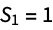, meaning you start with one dollar (tip: S stands for \$).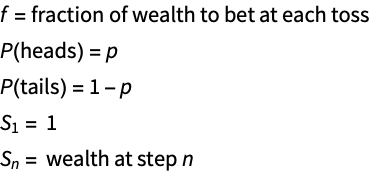Our goal is going to be to come up with a strategy that will maximize our long term wealth.

Coming up with a formula forThe first thing we need to do when confronted with a problem like this is to figure out a formula for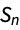. Note that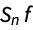is the amount that will be bet while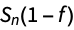is the amount that will not be bet.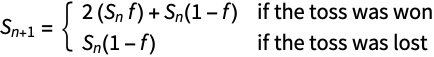Which we can re-write as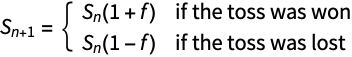Now, if we let W be the number of wins and L the number of loses,  we can simplify as follows: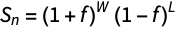Note: if you’re not sure why, just realize that your capital is either growing by (1+f) when you win or decreasing by (1-f) when you lose, now consider what happens if you win 2 times or lose 2 times, etc.

Now, notice that as n grows, the following becomes a good approximationWe can then replace W and L with our probabilities from theformula, which gives us our first achievement, a formula for, which is valid for large n.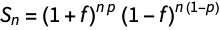Applying the Kelly Criterion for the first time

So we’ve found a closed formula that describes the gamblers wealth over time. We now want to, in some sense play around with f, which is really the only variable we can control and in some sense maximize.

From an intuitive perspective, we know that if f  is higher, we will win more over time (we assume that the bet is favorable, meaning p > 0.5), but we also know that if f is too high, we might go bust. Applying the Kelly Criterion will give us the right f so that we grow as fast as possible, while completely avoiding the risk of going bust.

Let’s introduce a number G called the rate of exponential growth, which we define as follows: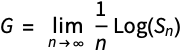Where the base of the logarithm is 2. To better understand G, let’s consider the case when the bets where totally guaranteed, meaning p=1. Then at each step, the wealth would double because you would bet everything you have. Then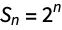, which plugging into the formula for G yields a value of G=1.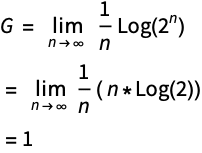So when G is 1, our capital double on every step. Let’s now try to find the general formula for G, using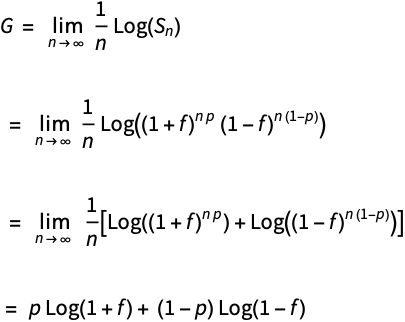Our goal, and the Kelly Criterion is going to be to maximize G, which in this context just means to find the value of f that maximizes G. Let’s plot G, as a function of f and p: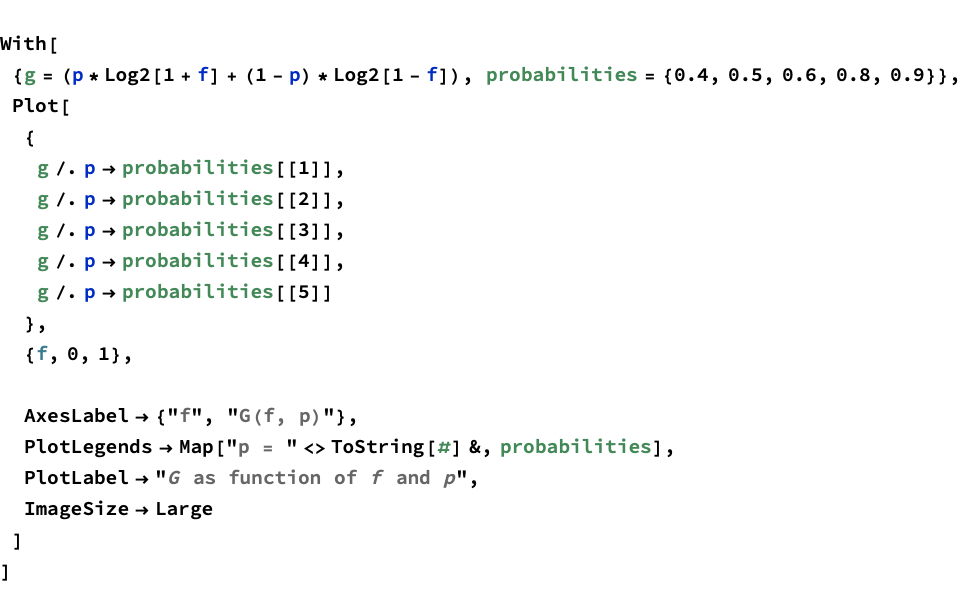Notice that when p is 0.4 G is 0. What this basically means is that you should never bet if the odds are against you. It might be hard to see, but when p is 0.5, Kelly is also telling us not to bet. As p becomes positive, we notice that a maximum does begin to emerge, when p is 0.9, Kelly is telling us to bet slightly more than half of our capital.

I won’t go too deep into the math, but it can be shown that G achieves its maximum when f=1-2p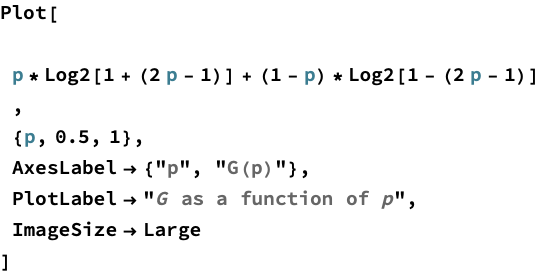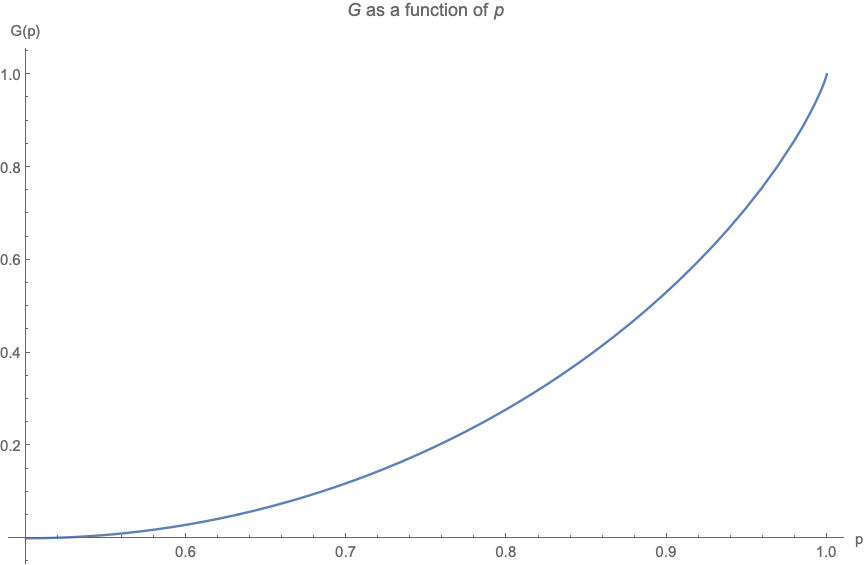Let’s end this chapter by showing you the results of simulating many Kelly gamblers with different values of f and noticing how their  wealth changes over time.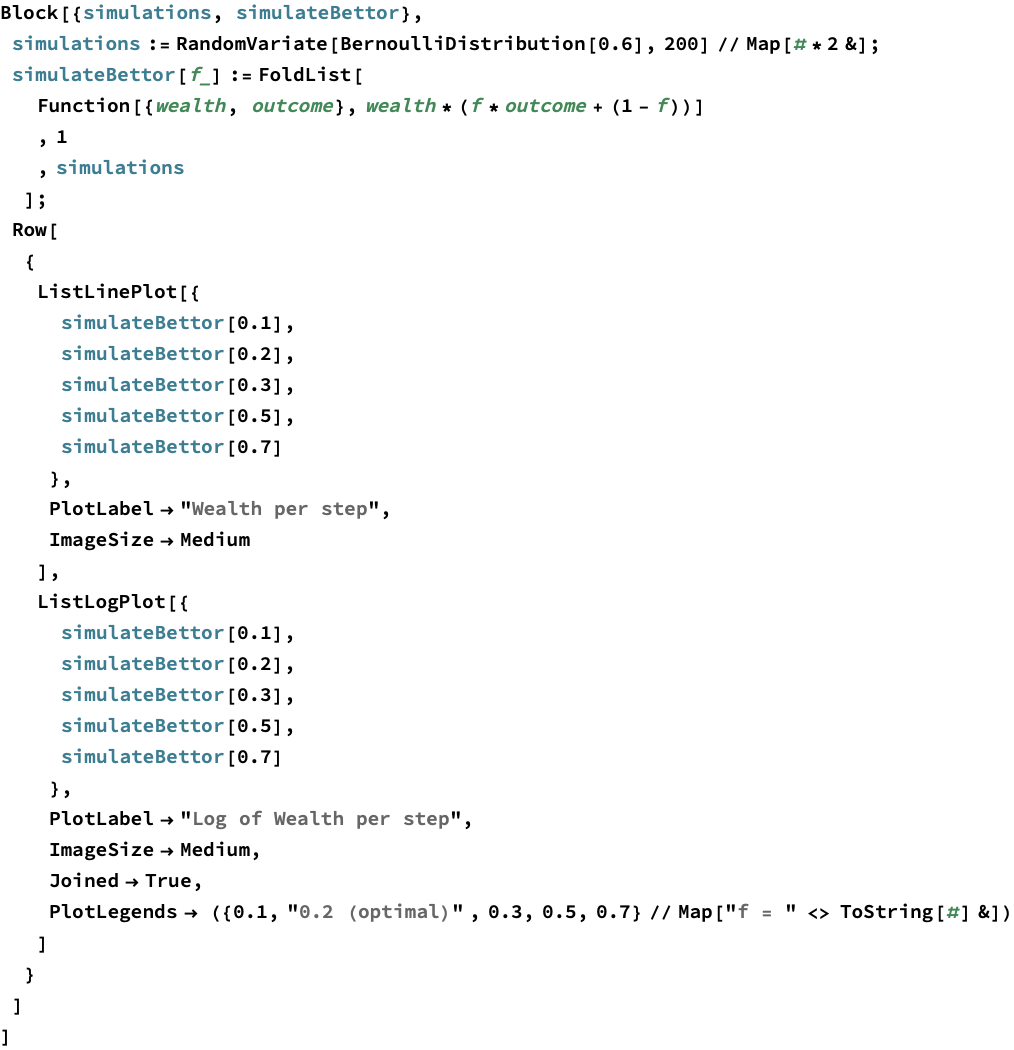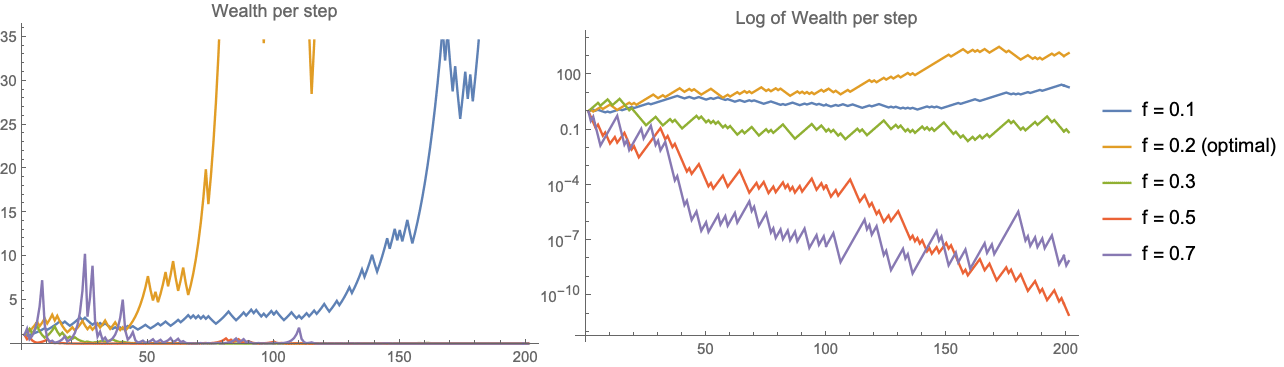On the left you see the wealth per step and to make it easier to understand the trends, the right shows the same plot on a logarithmic scale. What you will see is that the optimal bet size always ends up with the highest wealth over time and any bettor who bets more than Kelly ends up bust.

Conclusions

I hope this chapters gives you a first taste of what the Kelly Criterion is all about. We will be going deeper into the Kelly Criterion in future chapters, and we will try to apply the Kelly Criterion to situations more complicated than a coin toss. But for now let’s review the main points:

The Kelly Criterion is a technique to maximize long term wealth, when presented with an opportunity that has favorable odds.

The Kelly Criterion can be used to determine the maximum size of a bet.

Betting more than Kelly will lead you to bankruptcy.

Applying the Kelly Criterion means maximizingCheck Also
Close